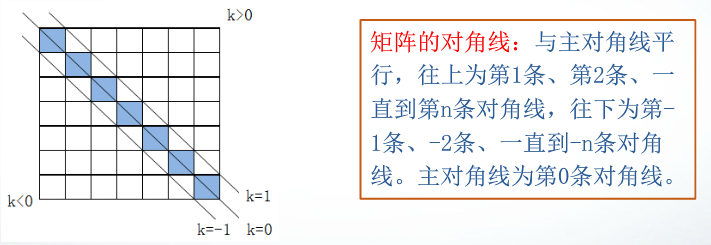• matlab提取矩阵对角元素
万次阅读 多人点赞
2019-01-04 17:19:00

对于提取矩阵的对角线，是很好办的，只需要一个函数就可以

diag(A);


那么怎么更改对角线上的数据呢？
可以借助对焦矩阵来解决：

A(logical(eye(size(A))))=B；


这里用eye函数生成和A一样的对角矩阵，然后使用logical函数来对A的对角线函数进行赋值。
这里B是对角线的元素组成的向量。

更多相关内容
• 关于matlab中的diag函数(矩阵对角元素提取和创建对角阵)diag函数功能：矩阵对角元素提取和创建对角阵设以下X为方阵，v为向量1、X = diag(v,k)当v是一个含有n个元素的向量时，返回一个n+abs(k)阶方阵X，向量v在...

关于matlab中的diag函数(矩阵对角元素的提取和创建对角阵)

diag函数功能：矩阵对角元素的提取和创建对角阵

设以下X为方阵，v为向量

1、X = diag(v,k)

当v是一个含有n个元素的向量时，返回一个n+abs(k)阶方阵X，向量v在矩阵X中的第k个对角线上，k=0表示主对角线，k>0表示在主对角线上方，k<0表示在主对角线下方。

例1：

v=[1 2 3];

diag(v, 3)

ans =

0 0 0 1 0 0

0 0 0 0 2 0

0 0 0 0 0 3

0 0 0 0 0 0

0 0 0 0 0 0

0 0 0 0 0 0

注：从主对角矩阵上方的第三个位置开始按对角线方向产生数据的

例2：

v=[1 2 3];

diag(v, -1)

ans =

0 0 0 0

1 0 0 0

0 2 0 0

0 0 3 0

注：从主对角矩阵下方的第一个位置开始按对角线方向产生数据的

2、X = diag(v)

向量v在方阵X的主对角线上，类似于diag(v,k),k=0的情况。

例3：

v=[1 2 3];

diag(v)

展开全文• 称呼： x = getIsub(X) 给定一个 SQUARE 矩阵 X，该函数提取对角元素的向量。matlab
• >> A = randi(3, 3)A = 3 3 1 1 2 2 3 3 31. diag(A)（尽可以查看，不可以修改）>> diag(A) ans = 3 2 32. 进行索引>> A(eye(size(A, 1)) == 1) ans = 3 2 3
>> A = randi(3, 3)

A =

3     3     1
1     2     2
3     3     3

## 1. diag(A)（尽可以查看，不可以修改）

>> diag(A)
ans =

3
2
3

## 2. 进行索引

>> A(eye(size(A, 1)) == 1)
ans =

3
2
3
展开全文• MATLAB基础二 首先产生5阶两位随机整数矩阵A，再产生均值为0.6、方差为0.1的5阶正态分布随机矩阵B，最后验证(A+B)I=IA+BI（I为单位矩阵）。 rand函数：产生（0，1）开区间均匀分布的随机数x。 fix(a+(b-a+1)*x)...

###MATLAB基础二

1. 首先产生5阶两位随机整数矩阵A，再产生均值为0.6、方差为0.1的5阶正态分布随机矩阵B，最后验证(A+B)I=IA+BI（I为单位矩阵）。
rand函数：产生（0，1）开区间均匀分布的随机数x
fix(a+(b-a+1)x)：产生[a,b]区间上均匀分布的随机整数。
randn函数：产生均值为0、方差为1的标准正态分布随机数
x*。
μ+σx：得到均值为μ、方差为σ²的随机数。

>>A=fix(10+(99-10+1)*rand(5))
A =
83 18 24 22 69
91 35 97 47 13
21 59 96 92 86
92 96 53 81 94
66 96 82 96 71
>> B=0.6+sqrt(0.1)randn(5)
B =
0.9272 0.8809 1.0549 0.5677 0.5905
0.8299 0.2373 0.7028 0.5236 0.5479
0.5040 0.2620 0.3613 0.7009 0.7985
0.6929 0.3440 1.0333 0.6989 0.9457
0.3510 -0.3311 0.0588 0.3265 0.9508
>> C=eye(5)
C =
1 0 0 0 0
0 1 0 0 0
0 0 1 0 0
0 0 0 1 0
0 0 0 0 1
>> (A+B)C==CA+B
C
ans =
1 1 1 1 1
1 1 1 1 1
1 1 1 1 1
1 1 1 1 1
1 1 1 1 1

1. 对角阵
（1）提取矩阵的对角线元素
diag(A)：提取矩阵A为主对角线元素，产生一个列向量。
diag(A,k)：提取矩阵A为第k条对角线元素，产生一个列向量。（2）构造对角阵
diag(V)：以向量V为主对角线元素，产生对角矩阵。
diag(V,k)：以向量V为第k条对角线元素，产生对角矩阵。

；提取对角阵
>>A =[
83 18 24 22 69;
91 35 97 47 13;
21 59 96 92 86;
92 96 53 81 94;
66 96 82 96 71];
>> diag(A)
ans =
83
35
96
81
71
>> diag(A,2)
ans =
24
47
86
；构造对角阵
>> diag(1:2:6)
ans =
1 0 0
0 3 0
0 0 5
>> diag(1:2:6,3)
ans =
0 0 0 1 0 0
0 0 0 0 3 0
0 0 0 0 0 5
0 0 0 0 0 0
0 0 0 0 0 0
0 0 0 0 0 0

1. 三角阵
（1）上三角阵
triu(A)：提取矩阵A的主对角线及以上的元素。
（2）下三角阵
函数为tril，其用法与triu相同。

>>triu(ones(4),-1)
ans =
1 1 1 1
1 1 1 1
0 1 1 1
0 0 1 1
>> tril(ones(4),-1)
ans =
0 0 0 0
1 0 0 0
1 1 0 0
1 1 1 0

1. 矩阵的转置
转置运算符是小数点后面接单引号（.’）。
共轭转置，其运算符是单引号（’），它在转置的基础上还要取每个数的复共轭。
即，复数情况下有区别。

2. 矩阵的旋转
rot90(A,k)：将矩阵A逆时针方向旋转90°的k倍，当k为1时可省略。

>>A =[
83 18 24 22 69;
91 35 97 47 13;
21 59 96 92 86;
92 96 53 81 94;
66 96 82 96 71]
>> rot90(A)
ans =
69 13 86 94 71
22 47 92 81 96
24 97 96 53 82
18 35 59 96 96
83 91 21 92 66
>> rot90(A,2)
ans =
71 96 82 96 66
94 81 53 96 92
86 92 96 59 21
13 47 97 35 91
69 22 24 18 83

1. 矩阵的翻转
对矩阵实施左右翻转是将原矩阵的第一列和最后一列调换，第二列和倒数第二列调换，…，以此类推。
fliplr(A)：对矩阵A实施左右翻转。
flipud(A)：对矩阵A实施上下翻转。

2. 矩阵的求逆
对于一个方阵A，如果存在一个与其同阶的方阵B，使得AB=BA=I（I为单位矩阵），则称B为A的逆矩阵，当然，A也是B的逆矩阵。
inv(A)：求方阵A的逆矩阵。

参考：MOOC《科学计算与MATLAB语言》

展开全文MATLAB 基础
• diag函数功能：矩阵对角元素提取和创建对角阵 设以下X为方阵，v为向量 1、X = diag(v,k)当v是一个含有n个元素的向量时，返回一个n+abs(k)阶方阵X，向量v在矩阵X中的第k个对角线上，k=0表示主对角线，k>0表示在...
• 我在应用程序中有一个小的性能瓶颈,需要从大的方阵中删除非对角元素.所以,矩阵x17 24 1 8 1523 5 7 14 164 6 13 20 2210 12 19 21 311 18 25 2 9变17 0 0 0 00 ...
• A = randi(5,5); % 随机生成每个元素1-5整数范围内的一个5*5的方阵 diag(A); %取主对角线上的元素 diag(A(1:end,end:-1:1)); % 取副对角线上的元素matlab 主对角线
• Matlab矩阵元素与子矩阵的提取 Matlab矩阵元素与子矩阵的提取 >> %矩阵元素提取 >> A=[1 2 3;4 5 6;7 8 9;10 11 12] A = 1 2 3 4 5 6 7 8 9 10 11 12 >> A(3,1)%提取A矩阵第3行，第1列的元素 ...
• 第2章 MATLAB矩阵及其运算 2.1 变量和数据操作 2.2 MATLAB矩阵 2.3 MATLAB运算 2.4 矩阵分析 2.5 矩阵的超越函数 2.6 字符串 2.7 结构数据和单元数据 2.8 稀疏矩阵 2.1 变量和数据操作 2.1.1 变量与赋值 1....
• 学习笔记若有错误请指正QAQ ...矩阵的对角元素提取 有一个N*N的W矩阵提取其中对角线的值 首先建立一个N*N的0矩阵D，之后使用for循环将W矩阵对角线上的值赋给D矩阵 D=zeros(N); for k=1:N D(k,k)=W(...
• 1、对角阵 （1）提取矩阵对角线元素 >> a = [1:3;4:6;7:9] a = 1 2 3 4 5 6 7 8 9 >> diag(a) ans = 1 5 .matlab
• Matlab 中的 diag(M) 以某种方式将对角线提取为并非总是必要的向量matlab
• 构造其对角矩,matlab 产生一个三行三列随机矩阵矩阵的元素范围012019-4-13来自ip:16.165.132.189的网友咨询浏览量:373手机版问题补充：用matlab 生成一个3X3随机矩阵A,提取对角元素,构造其对角矩阵...,matlab ...
• 我编写了一个代码来生成一个包含四列的矩阵,以获得总和等于9的所有数字组合,每个数字从0到9不等.m = zeros(220, 4);pd = 9;i = 1;for p = 0:1:pdfor q = 0:1:pd-pfor a = 0:1:pd-q-pm(i,:) = [p, q, a, pd-a-q-p];i ...
• 如何用MATLAB随机提取矩阵的100行，并使剩下的构成新矩阵构成matlab
• 二、MATLAB矩阵处理 2.1 特殊矩阵 通用的特殊矩阵：zero()产生0矩阵，one()全1矩阵，eye()产生对角线为1的矩阵，MATLAB()产生(0...提取矩阵对角线元素：diag(A,k=0)：提取矩阵A第k条元素，产生列向量 构造对角矩阵：dia
• 稀疏矩阵：是指零元素的个数远远多于非零元素的个数的矩阵 1、矩阵的存储方式 （1）完全存储方式 （2）稀疏存储方式 稀疏存储方式只存储矩阵的非零元素的值及其位置,即行号和列号。 注意,采用稀疏存储方式时，矩阵...matlab
• 今天我把之前学习以及用到的关于用MATLAB读写Excel数据，并进行计算处理的经验分享给需要的小伙伴。参加过数学建模的这个应该就很简单了，不经常使用的来说可能有点麻烦。小编这几天学习使用MATLAB也走了一些弯路...
• triu() 函数是 中提取上三角矩阵的函数，其调用形式如下：U=triu(X)或者U=triu(X,k)其中，X 是待求解的数组，前者函数返回的结果，为数组 X 的主对角线及其右上方的数，且其余位置上的元素为 0。后者函数返回的结果...
• 目录对角阵与三角阵1、对角阵（1）提取矩阵对角线元素(2)构造对角线 2、三角阵(1)上三角阵(2)下三角阵矩阵的转置与旋转1、矩阵的转置2、矩阵的旋转3、矩阵的左右翻转4、矩阵的上下翻转矩阵的逆与伪逆1、矩阵的逆 ...matlab
• (1)提取矩阵对角线元素 diag(x)提取主对角线上的元素,产生一个列向量 diag(x,k)提取第K条对角线的元素,产生一个列向量 (2)构造对角矩阵 diag(v):以向量v为主对角线元素,产生对角矩阵 diag(v,k):以向量v为第k条...matlab
• 包括构造稀疏的离散微分几何算子和有限元矩阵，例如切线拉普拉斯和对角化质量矩阵，简单的基于面和边的拓扑数据结构，用于OpenGL和GLSL的网格查看实用程序以及许多核心功能矩阵处理函数，使您感觉更像MATLAB。...
• Matlab(12)——稀疏矩阵文章目录Matlab(12)——稀疏矩阵一、矩阵的...带状稀疏矩阵的稀疏存储方式①从带状稀疏矩阵提取对角线上元素②创建带状稀疏矩阵4.单位矩阵的稀疏存储方式一、矩阵的存储方式1.完全存储方式...
• 矩阵同一行中的元素之间用 空格 或 逗号 分隔 矩阵行与行之间用 分号 分开 直接输入法中，分号可以用 回车 代替 A=[1 2 3 4 5 6 7 8 9;1 2 3] 利用MATLAB函数创建矩阵 基本矩阵函数如下： (1) ones()函数：产生全为...
• eye函数：产生对角线为1的矩阵。当矩阵是方阵时，得到一个单位矩阵。 rand函数：产生（0，1）区间均匀分布的随机矩阵。 randn函数：产生均值为0，方差为1的标准正态分布随机矩阵。 zeros函数的调用格式： zeros(m)：...matlab
• eye函数：产生对角线为1的矩阵矩阵是方阵时，得到一个单位矩阵 rand函数：产生（0,1）区间均匀分布的随机矩阵 randn函数：产生均值为0，方差为1的标准正态分布随机矩阵 调用格式： zeros(m):产生m *m零矩阵 zeros...
• 即可输出disp(a) Matlab常用生成矩阵函数： zeros(m,n) 生成一个 m 行 n 列的零矩阵，m=n 时可简写为 zeros(n) ones(m,n)生成一个 m 行 n 列的元素全为 1 的矩阵, 当m=n 时可写为 ones(n) eye(m,n) 生成一个主对角线...
• 1.3 对角元素矩阵 例题1 （1）由行向量生成对角矩阵 （2）由列向量通过转置变成行向量 （3）主对角线上面第k条对角线为向量C的矩阵 例题2 二. Hilbert矩阵及逆Hilbert矩阵 三. Hankel(汉克）矩阵 四. ...matlab
• 相关问题:匿名网友:matlab中取一个矩阵的一部分方法如下：格式A(m,n)，用于提取矩阵A中符合m,n要求的部分1、提取某个元素，则m,n为数字标量，如A(2,3)为第二行第三列的元素。2、提取某行某列A(：，n)提取第......